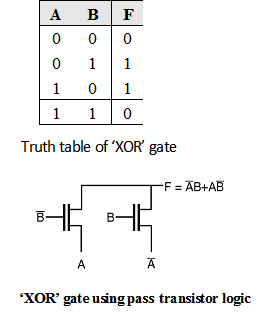Home > Digital CMOS Design > Pass Transistor Logic > XOR gate using pass transistor logic

Pass-Transistor-Logic

'XOR' gate using pass transistor logic.

The truth table of 'XOR' gate is as shown in Table below. Figure below shows the implementation of 'XOR' function using pass transistors. In this gate if the B input is low then left NMOS transistor is ON and the logic value of A is copied to the output F. When B input is high right NMOS transistor is ON and the inverted logic value of A is copied to the output F, which satisfies the truth table of the XOR gate.